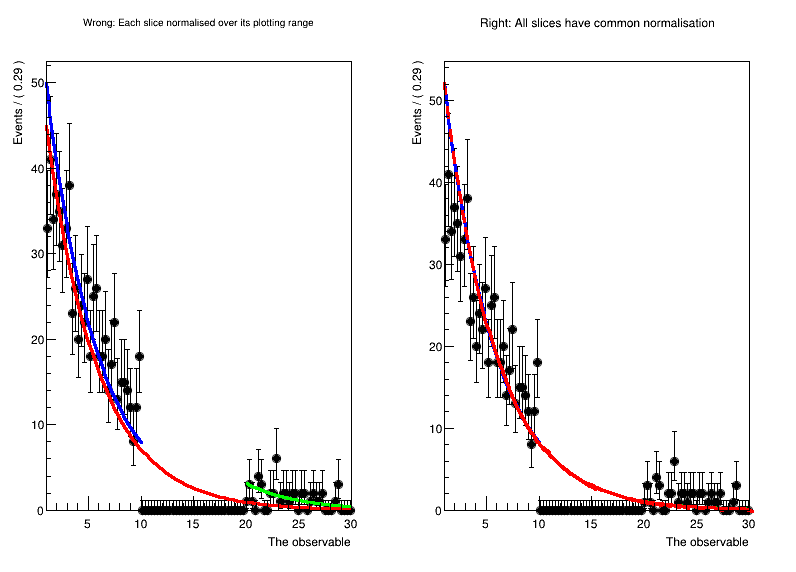# Rf 2 1 2_Plotting In Ranges_Blinding¶

Plot a PDF in disjunct ranges, and get normalisation right.

Usually, when comparing a fit to data, one should first plot the data, and then the PDF. In this case, the PDF is automatically normalised to match the number of data events in the plot. However, when plotting only a sub-range, when e.g. a signal region has to be blinded, one has to exclude the blinded region from the computation of the normalisation.

In this tutorial, we show how to explicitly choose the normalisation when plotting using NormRange().

Thanks to Marc Escalier for asking how to do this correctly.

Author: Stephan Hageboeck
This notebook tutorial was automatically generated with ROOTBOOK-izer from the macro found in the ROOT repository on Thursday, December 09, 2021 at 09:35 AM.

In :
%%cpp -d
#include <RooDataSet.h>
#include <RooExponential.h>
#include <RooPlot.h>
#include <RooRealVar.h>
#include <TCanvas.h>

In :
%%cpp -d
// This is a workaround to make sure the namespace is used inside functions
using namespace RooFit;

In :
// Make a fit model
RooRealVar x("x", "The observable", 1, 30);
RooRealVar tau("tau", "The exponent", -0.1337, -10., -0.1);
RooExponential exp("exp", "A falling exponential function", x, tau);

// Define the sidebands (e.g. background regions)
x.setRange("full", 1, 30);
x.setRange("left", 1, 10);
x.setRange("right", 20, 30);

// Generate toy data, and cut out the blinded region.
RooDataSet* data = exp.generate(x, 1000);
auto blindedData = data->reduce(CutRange("left,right"));

// Kick tau a bit, and run an unbinned fit where the blinded data are missing.
// ----------------------------------------------------------------------------------------------------------
tau.setVal(-2.);
exp.fitTo(*blindedData);

// Here we will plot the results
TCanvas *canvas=new TCanvas("canvas","canvas",800,600);
canvas->Divide(2,1);

// Wrong:
// Plotting each slice on its own normalises the PDF over its plotting range. For the full curve, that means
// that the blinded region where data is missing is included in the normalisation calculation. The PDF therefore
// comes out too low, and doesn't match up with the slices in the side bands, which are normalised to "their" data.
// ----------------------------------------------------------------------------------------------------------

std::cout << "Now plotting with unique normalisation for each slice." << std::endl;
canvas->cd(1);
RooPlot* plotFrame = x.frame(RooFit::Title("Wrong: Each slice normalised over its plotting range"));

// Plot only the blinded data, and then plot the PDF over the full range as well as both sidebands
blindedData->plotOn(plotFrame);
exp.plotOn(plotFrame, LineColor(kRed),   Range("full"));
exp.plotOn(plotFrame, LineColor(kBlue),  Range("left"));
exp.plotOn(plotFrame, LineColor(kGreen), Range("right"));

plotFrame->Draw();

// Right:
// Make the same plot, but normalise each piece with respect to the regions "left" AND "right". This requires setting
// a "NormRange", which tells RooFit over which range the PDF has to be integrated to normalise.
// This is means that the normalisation of the blue and green curves is slightly different from the left plot,
// because they get a common scale factor.
// ----------------------------------------------------------------------------------------------------------

std::cout << "\n\nNow plotting with correct norm ranges:" << std::endl;
canvas->cd(2);
RooPlot* plotFrameWithNormRange = x.frame(RooFit::Title("Right: All slices have common normalisation"));

// Plot only the blinded data, and then plot the PDF over the full range as well as both sidebands
blindedData->plotOn(plotFrameWithNormRange);
exp.plotOn(plotFrameWithNormRange, LineColor(kBlue),  Range("left"),  RooFit::NormRange("left,right"));
exp.plotOn(plotFrameWithNormRange, LineColor(kGreen), Range("right"), RooFit::NormRange("left,right"));
exp.plotOn(plotFrameWithNormRange, LineColor(kRed),   Range("full"),  RooFit::NormRange("left,right"), LineStyle(10));

plotFrameWithNormRange->Draw();

canvas->Draw();RooFit v3.60 -- Developed by Wouter Verkerke and David Kirkby
Copyright (C) 2000-2013 NIKHEF, University of California & Stanford University

[#1] INFO:Eval -- RooRealVar::setRange(x) new range named 'full' created with bounds [1,30]
[#1] INFO:Eval -- RooRealVar::setRange(x) new range named 'left' created with bounds [1,10]
[#1] INFO:Eval -- RooRealVar::setRange(x) new range named 'right' created with bounds [20,30]
[#1] INFO:Minimization -- RooAbsMinimizerFcn::setOptimizeConst: activating const optimization
**********
**    1 **SET PRINT           1
**********
**********
**********
PARAMETER DEFINITIONS:
NO.   NAME         VALUE      STEP SIZE      LIMITS
1 tau         -2.00000e+00  9.50000e-01   -1.00000e+01 -1.00000e-01
**********
**    3 **SET ERR         0.5
**********
**********
**    4 **SET PRINT           1
**********
**********
**    5 **SET STR           1
**********
NOW USING STRATEGY  1: TRY TO BALANCE SPEED AGAINST RELIABILITY
**********
**********
FIRST CALL TO USER FUNCTION AT NEW START POINT, WITH IFLAG=4.
START MIGRAD MINIMIZATION.  STRATEGY  1.  CONVERGENCE WHEN EDM .LT. 1.00e-03
FCN=6752.91 FROM MIGRAD    STATUS=INITIATE        4 CALLS           5 TOTAL
EDM= unknown      STRATEGY= 1      NO ERROR MATRIX
EXT PARAMETER               CURRENT GUESS       STEP         FIRST
NO.   NAME      VALUE            ERROR          SIZE      DERIVATIVE
1  tau         -2.00000e+00   9.50000e-01   2.51381e-01  -1.27116e+04
ERR DEF= 0.5
MIGRAD WILL VERIFY CONVERGENCE AND ERROR MATRIX.
COVARIANCE MATRIX CALCULATED SUCCESSFULLY
FCN=1941.97 FROM MIGRAD    STATUS=CONVERGED      30 CALLS          31 TOTAL
EDM=3.68147e-07    STRATEGY= 1      ERROR MATRIX ACCURATE
EXT PARAMETER                                   STEP         FIRST
NO.   NAME      VALUE            ERROR          SIZE      DERIVATIVE
1  tau         -2.04501e-01   7.81359e-03   2.33650e-04  -7.85653e-02
ERR DEF= 0.5
EXTERNAL ERROR MATRIX.    NDIM=  25    NPAR=  1    ERR DEF=0.5
6.105e-05
**********
**    7 **SET ERR         0.5
**********
**********
**    8 **SET PRINT           1
**********
**********
**    9 **HESSE         500
**********
COVARIANCE MATRIX CALCULATED SUCCESSFULLY
FCN=1941.97 FROM HESSE     STATUS=OK              5 CALLS          36 TOTAL
EDM=3.6779e-07    STRATEGY= 1      ERROR MATRIX ACCURATE
EXT PARAMETER                                INTERNAL      INTERNAL
NO.   NAME      VALUE            ERROR       STEP SIZE       VALUE
1  tau         -2.04501e-01   7.81358e-03   4.67300e-05   1.36495e+00
ERR DEF= 0.5
EXTERNAL ERROR MATRIX.    NDIM=  25    NPAR=  1    ERR DEF=0.5
6.105e-05
[#1] INFO:Minimization -- RooAbsMinimizerFcn::setOptimizeConst: deactivating const optimization
Now plotting with unique normalisation for each slice.
[#0] WARNING:Plotting -- Cannot apply a bin width correction and use Poisson errors. Not correcting for bin width.
[#1] INFO:Plotting -- RooAbsPdf::plotOn(exp) only plotting range 'full', curve is normalized to data in given range
[#1] INFO:Plotting -- RooAbsPdf::plotOn(exp) only plotting range 'left', curve is normalized to data in given range
[#1] INFO:Plotting -- RooAbsPdf::plotOn(exp) only plotting range 'right', curve is normalized to data in given range

Now plotting with correct norm ranges:
[#0] WARNING:Plotting -- Cannot apply a bin width correction and use Poisson errors. Not correcting for bin width.
[#1] INFO:Plotting -- RooAbsPdf::plotOn(exp) only plotting range 'left'
[#1] INFO:Plotting -- RooAbsPdf::plotOn(exp) p.d.f. curve is normalized using explicit choice of ranges 'left,right'
[#1] INFO:Plotting -- RooAbsPdf::plotOn(exp) only plotting range 'right'
[#1] INFO:Plotting -- RooAbsPdf::plotOn(exp) p.d.f. curve is normalized using explicit choice of ranges 'left,right'
[#1] INFO:Plotting -- RooAbsPdf::plotOn(exp) only plotting range 'full'
[#1] INFO:Plotting -- RooAbsPdf::plotOn(exp) p.d.f. curve is normalized using explicit choice of ranges 'left,right'


Draw all canvases

In :
%jsroot on
gROOT->GetListOfCanvases()->Draw()